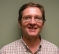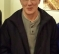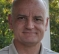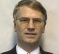## fft process gain revisitedStarted by 7 months ago22 replieslatest reply 6 months ago465 views

Hi All,

if you google "fft process gain" you get plenty hits including various analysis down to bin spread concepts.

However, I notice that this can be viewed in a much simpler way:

If we force fft output of a single tone to be equal to its input then the gain is removed and it  is so irrespective of fft resolution. So the fft process gain is just due to arbitrary fft scaling (by most tools as well fft formula).

With due respect, I see the popular analysis as not helpful conceptually.

example:

%%%%%%%%%%%%%%%%%%%%%%%%%%%

x = round(2^15*exp(j*2*pi*(0:2047)*.1)); %single tone

y1 = fft(x);

y2 = fft(x)/sqrt(2048);

px = 10*log10(mean(abs(x).^2));

p1 = 10*log10(mean(abs(y1).^2));

p2 = 10*log10(mean(abs(y2).^2));

[px, p1, p2] %input pwr, fft output pwr, fft descaled

[ - ]I think you've missed the point of what "process gain" means.  It is independent of scaling.

Filters generally give SNR gain by removing the noise in the stopbands, and decimators also give a reduction in quantization noise, so a quantifiable gain in dynamic range.  A correlator has process gain by rejecting non-correlated signals and amplifying correlated signals.

An FFT bin can be viewed as a filter, where the bandwidth is 1/N of the supported bandwidth, where N is the length of the FFT, or as a correlator, where the correlation reference function is the basis function for the transform (in this case a sinusoid).  The gain for that is also proportional to N, so it is consistent with the filter bandwidth viewpoint.

All of those process gains are independent of scaling.

[ - ]Exactly.

If you consider white noise power to be evenly distributed in the Nyquist zone then your processing gain is the Nyquist bandwidth / equivalent noise power bandwidth of an FFT bin including any windowing effects.

If I remove the noise from 1/2 of the Nyquist bandwidth (say a sharp half band filter) then 1/2 of the noise power is gone.  That is a 3dB noise gain.

[ - ]Yes that is true for Nyquist but I am focused on FFT as a process independant of sampling rate, just the mathematical fft operation.

[ - ]Thanks for the response.

I see you are looking at SNR.

The snr formula for the fft gain effect is extra 10log(N/2). If same fft resolution is descaled for power unity then above formula doesn't apply any more.

I am aware that if I apply 4k resolution I will spread bin power on twice as many bins as 2k fft. I am comparing 2k case only (standard fft vs unity power fft)

When I look at spectrum "peak to floor" I don't see much difference between fft and its scaled down version.

%%%%%%%%%%%%%%%%%%%%%%%%%%%%%%%%%%%%%%%%%%%%%%%%%%%%%

x = round(2^15*exp(j*2*pi*(0:2047)*.1));

y1 = fft(x);

y2 = round(fft(x)/sqrt(2048));

y1b = y1/max(abs(y1));

y2b = y2/max(abs(y2));

plot(10*log10(abs(y1b).^2))

hold

plot(10*log10(abs(y2b).^2),'r--')

[ - ]Parseval's theorem says the total power in and out will be the same in a linear transform.   So if you scale them so that the total power is the same, fine, that's one way to scale it.

But the process gain of an FFT bin is still proportional to N, and still the usual formula.   I think you just don't quite get the idea yet.

If you're looking for a tone that's kind of stuck in noise and difficult to discern in the time domain, it'll pop up WAY HIGH in its FFT bin above the other bins.   That's process gain.

[ - ]Slartibartfest-

"difficult to discern" -- some years ago when I would hire new DSP and software engineers I would pull up Hypersignal and display a huge speech waveform, zoom in, grab one point, and move it slightly -- not a lot, just a tad. Then I'd zoom out and challenge the engineer: find that point.  Some would try for a while by zooming and panning around in the time display, but of course it was undiscernable. Then we would do an FFT with 50% overlap and windowing and look at the 2-D spectrograph. Aha, what's that vertical line doing there ?

I will never forget how many times the newbies would look at me wide-eyed and say something like, dang we studied FFTs but I never realized it was that powerful.  Now *that* is process gain.

-Jeff

[ - ]Post deleted by author

[ - ]There seems to be two perspectives:

1)Processing gain: For bin resolution a higher N gives better insight and spreads same power across more bins leading to false higher SNR by 10log(N/2) This applies to a sampling ADC device.

2)Pseudo gain: Within digital domain, comparing N size fft on same single tone vector using standard fft versus unity power. Standard fft gives 10log(N/2) extra SNR as in 1) above. The same fft scaled down for power unity gives no false SNR. This is my original assumption and so I should have named the title better e.g. pseudo process gain.

As FPGA engineer I am stuck to digital vectors but most DSP concepts are centered on ADC/DAC.

Here is an excerpt from: [Taking the Mystery out of the Infamous Formula, "SNR = 6.02N + 1.76dB," and Why You Should Care by Walt Kester]

"Figure 6 shows the FFT output for an ideal 12-bit ADC. Note that the average value of the noise floor of the FFT is approximately 107 dB below full-scale, but the theoretical SNR of a 12-bit ADC is 74 dB. The FFT noise floor is not the SNR of the ADC, because the FFT acts like an analog spectrum analyzer with a bandwidth of fs/M, where M is the number of points in the FFT. The theoretical FFT noise floor is therefore 10log10(M/2) dB below the quantization noise floor due to the processing gain of the FFT. "

edit: by the way another weird thing with above ADC example:

what should N be to remove the false gain?

10log10(N/2) to be 0

hence N = 2

[ - ]There is no "false gain".   I think there is just a gap in your understanding of what people mean by "processing gain".

[ - ]ok theoretical gain instead of false gain.

In the 12 bits ADC example the theoretical SNR should be 74dB but it is 107 as seen on given FFT.

[ - ]You have to account for everything that is going on.   There is processing gain in the FFT as well as an increase in dynamic range by suppressing the quantization noise.  In other words, after the FFT filtering there are more significant bits than when you started, so the 74dB limitation of 12 bits no longer applies.   There's no mystery, you just have to understand it all.

[ - ]I understand your view. But the output from 12 bits ADC is 12 bits to me and I should not assume I will replace it with fft output for further processing. I just wanted fft to measure SNR which originally is 74dB only.

Let me model the above ADC single tone example (from Walt Kester), roughly: If I rescale the fft output it behaves.

%%%%%%%%%%%%%%%%%%%%%%%%%%%%%%%%%%%%%%%%%%%%%%%%%

x = round(2^12*exp(j*2*pi*(0:4095)*.1)); %sampled at ADC

y1 = fft(x);

y2 = fft(x)/sqrt(4096);

px = 10*log10(mean(abs(x).^2));

p1 = 10*log10(mean(abs(y1).^2));

p2 = 10*log10(mean(abs(y2).^2));

round([px,p1,p2])     %dB in each case

y1b = y1/max(abs(y1));

y2b = y2/max(abs(y2));

plot(10*log10(abs(y1b).^2))

hold

plot(10*log10(abs(y2b).^2),'r--')

[ - ]A noise density is an easier way I think to view this, as the noise density (in power/Hz specifically NOT power/bin) for spread waveforms (such as quantization noise) does not change. What changes with the FFT is the resolution bandwidth which will be very clear for anyone that has worked with a spectrum analyzer. The equivalent noise bandwidth in Hz of the unwindowed FFT (no additional shaped window beyond the rectangular window given by the data selection) is simply the sampling rate in Hz divided by the number of bins, thus if we increase the number of bins, any spread noise such as quantization noise will go down as the power within the bandwidth of each bin is less. (We see the same thing when adjusting the RBW on a spectrum analyzer).

That formula mentioned for ADC SNR predicts the total power over the entire first Nyquist zone as a two-sided power spectral densit (or DC to the sampling rate), which is spread evenly in frequency as white noise (to a very good approximation for an uncorrelated sampling clock), so the relationship with resolution bandwidth and it’s implied “processing gain” works quite well in that case.

Where it gets really interesting is when windowing is applied. This is specifically where I have seen the  “processing gain” applied in a meaningful way as I detail at this Stack Exchange post: https://dsp.stackexchange.com/questions/75817/converting-from-psd-v2-hz-to-dbv-1-volt-rms-reference/75823#75823  Windowing in time increases the resolution bandwidth to be greater than one bin, such that the power represented in each bin is overlapping with that in adjacent bins. This results in a different estimate or power for spectrally pure tones versus spread waveforms, but is well analyzed from the processing gain which is the ratio of the coherent gain of the window as given my its mean to the non coherent gain as given my its standard deviation. The inverse of the processing gain squared is the resolution bandwidth for each bin

[ - ]I agree with bin power spread concepts. That is not what I am focused on. I am focused on the fft as a math function away from sampling concepts, for single tone case containing only quantisation noise.

If Walt Kester rescaled his 4k fft by 1/sqrt(4096) his argument does not apply anymore as this scale factor is equivalent to -10log(N/2) so the same 4k fft will show correct QSNR:

Here is his statement again:

"... The theoretical FFT noise floor is therefore 10log10(M/2) dB below the quantization noise floor due to the processing gain of the FFT. "

[ - ]Sorry for my distraction about bin power spread due to windowing which is my preferred use of processing gain in an FFT. My bigger point was there is no actual change in SNR when we don’t use windowing as the noise density doesn’t change— so within any interval of bandwidth assuming both the signal and the noise have a bandwidth spread across multiple bins, the SNR is unchanged- we just see a portion of both in any bin. The FFT, like a bandpass filter (which it is: a bank of filters) can remove noise out of band, but that does not change the noise in band (the power spectral density of the noise is not changed).

The factor of two is specifically due to using a one-sided noise spectrum instead of two-sided. As long as we dictate which we use, that “Processing Gain” which is simply the portion of the same noise density due to quantization noise is either 10Log(N) or 10Log(N/2). If you use a two-sided noise density, it would be 10Log(N), and if you use a one-sided noise density, it would be 10Log(N/2).

I work more often with complex baseband signals where the FFT represents a unique spectrum from DC to the sampling rate. In this case a two-sided noise density is typically used and the “processing gain” is 10Log(N). The PSD for real waveforms can be presented with either one-sided or two-sided spectrum, so this is a detail that needs to be clarified on the PSD.

Note too the similarity with “Processing Gain” as used in direct sequence spread spectrum which is 10Log(N) for the same reason, simply a ratio of BW: here the bandwidth in the full Nyquist frequency range to the bandwidth of one bin.

[ - ]I have believed for a long time that the effective quantization noise in individual bins after an FFT is > the quantization noise in the time domain signal.
In OFDM, let's assume that I have 10 good bits per I and Q samples from the A/Ds, and then I take a 1024 point FFT.  Assume that the FFT creates no loss of precision during any of the intermediate stages.

How many good bits are there in the individual bins?

I ask, because I find that a receiver, that I am working with, only gives me the same number of bits per bin, in the FFT output, as there are in the A/D, which I think is depriving me of signal quality.

Thanks for any insight.

David

[ - ]Hi David,

Let me first correct my conclusions in this thread.

If FFT is not scaled down then it gives total power as (scaled input power) and gives SNR as (input SNR + processing gain).

if FFT is down scaled for unity it gives total power as (input power), but actual SNR stays as (input SNR + processing gain).

Above conclusions based on checking noise floor in spectrum using single tone that did not smear around. (both QSNR and added random noise checked).

So I now correct myself and agree with the views presented here that processing gain as SNR does not care about scaling.

The next question is why this gain does not apply to iFFT. iFFT gives us our input back exactly. Yet both FFT and iFFT are just different by the sign of frequency terms and some research corners actually use these terms in reverse of DSP (I think).

[ - ]This does apply equally in reverse, and you are correct that the operations are symmetric other than the sign on the exponential. Further by convention we scale the inverse by $1/N$ but there are forms where for each a scaling of $1/\sqrt{N}$ are applied. Scaling does not matter at all when we are concerned about SNR, but scaling is critical when we are mapping our results into a specific range (such as with fixed point, or where actual full scale is on an ADC or DAC).

So in the time domain if we had $e^{j \omega_1 t}$ (if you prefer to think in terms of a sine wave we can do that instead but I find the single exponential tone much more intuitive once you get used to it), we note that in the time domain it's representation is spread across every sample, as is the noise that we also add. When we convert this to the frequency domain, it's representation is condensed to just one sample (two if you are using a sinusoid) while the noise is still spread across every sample. This should reconcile your concern with the consistency of SNR when moving across both domains.

[ - ]Thanks Dan,

If we apply FFT followed by iFFT we get original input exact. So it is same original SNR and can't be anything else.

[ - ]Yes, no disagreement with that. I was trying to provide an explanation how it is the same and yet the same processing gain concepts apply (but apparently I didn't explain very well): in reverse there is only one signal sample in the frequency domain contributing to the time domain signal but all noise samples in the frequency domain contribute to the time domain noise, thus we get a "reverse processing gain" when we go back from frequency to time. Does that make more sense?

The signal frequency domain sample is spread across all time domain samples, while the noise in frequency which is across all samples is mapped to noise in time across all samples. In both cases we follow Parseval's Theorem, it is just how the energy is mapped that makes it different.

[ - ]Hi David-

The FFT is a narrow band filter, and the quantization noise (up to the limits of the SFDR) is spread evenly across all bins, so you can get a lot more bits of resolution in any one bin. If you look at the formula for the DFT and to simplify just consider the $k=0$ bin, you will see that $k=0$ is simply the average of the input samples (if we divided by $N$ which is just a scaling). If you average all the quantization noise in all $N$ samples, the standard deviation in the sum goes down by $1/\sqrt{N}$ which leads to the increase in precision. This is consistent with the "processing gain" as referenced here since each bit of resolution gives us 6 dB in SNR.

[ - ]What do figures say? After modelling I got these:

1) single complex tone, 12 bits signed, 2k FFT:

input:                QSNR: 74 dB

fft:                  QSNR: 106.6 dB, mean power: 99.3 dB

scaled fft for unity: QSNR: 106.4 dB, mean power: 66.2 dB

Hence fft process gain for SNR is maintained as ~+33 dB (scaled or not)

2) ofdm 20MHz (12 bits signed, 2k FFT):

input:                QSNR: 63.16 dB

fft:                  QSNR: 66.56 dB, mean power: 89.5 dB

scaled fft for unity: QSNR: 66.97 dB, mean power: 56.4 dB

Hence fft process gain is only ~+3 dB (scaled or not)

Thus FFT gain is independent of scaling yes, but its formula applies to single tone and much much less on band limited signal.

[ - ]